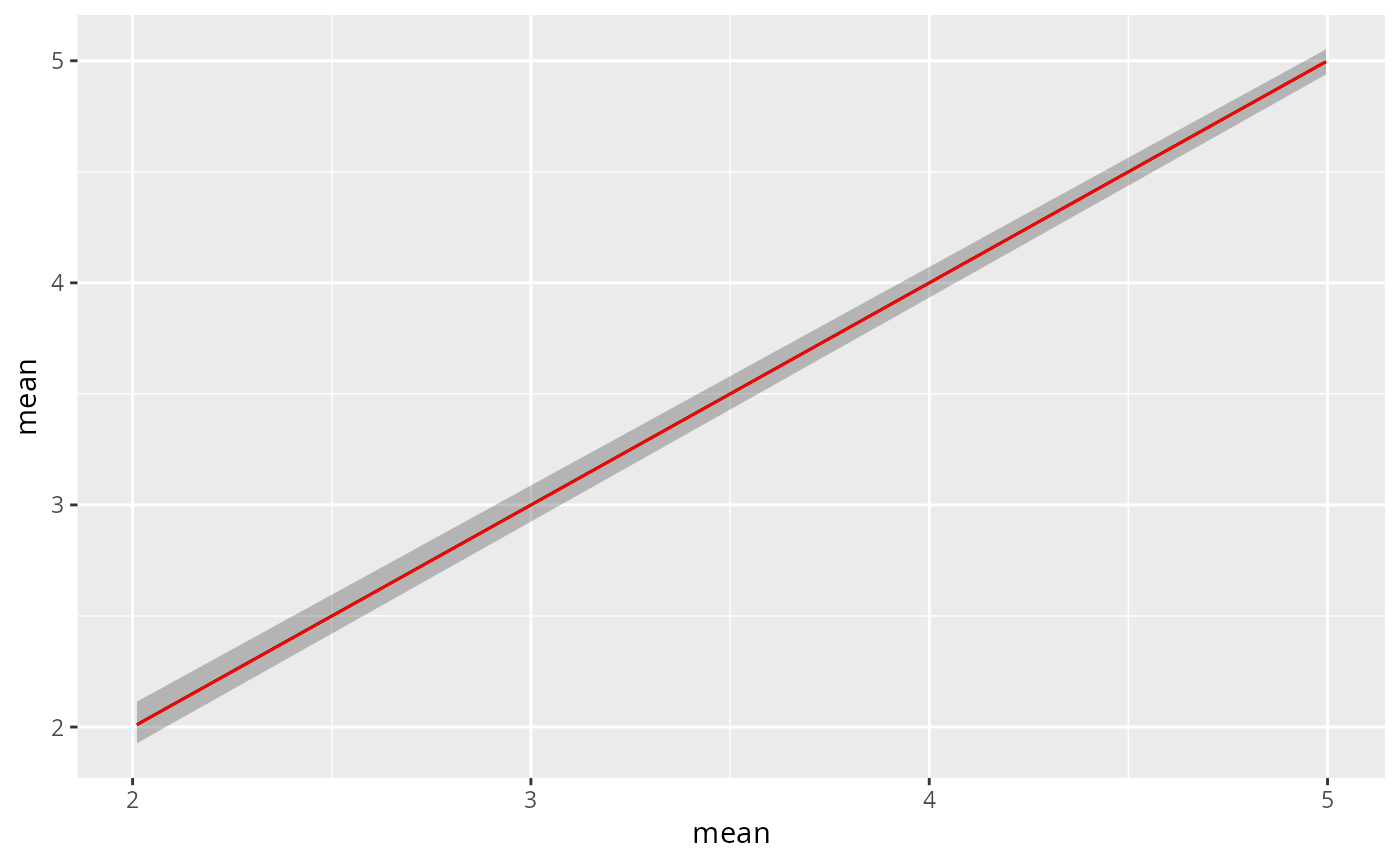This geom serves to visualize prediction objects which usually results from a call to predict.bru(). Predictions objects provide summary statistics (mean, median, sd, ...) for one or more random variables. For single variables (or if requested so by setting bar = TRUE), a boxplot-style geom is constructed to show the statistics. For multivariate predictions the mean of each variable (y-axis) is plotted agains the row number of the varriable in the prediction data frame (x-axis) using geom_line. In addition, a geom_ribbon is used to show the confidence interval.

Note: gg.bru_prediction also understands the format of INLA-style posterior summaries, e.g. fit$summary.fixed for an inla object fit Requires the ggplot2 package. ## Usage # S3 method for bru_prediction gg(data, mapping = NULL, ribbon = TRUE, alpha = NULL, bar = FALSE, ...) # S3 method for prediction gg(data, ...) ## Arguments data A prediction object, usually the result of a predict.bru() call. mapping a set of aesthetic mappings created by aes. These are passed on to geom_line. ribbon If TRUE, plot a ribbon around the line based on the smalles and largest quantiles present in the data, found by matching names starting with q and followed by a numerical value. inla()-style numeric+"quant" names are converted to inlabru style before matching. alpha The ribbons numeric alpha (transparency) level in [0,1]. bar If TRUE plot boxplot-style summary for each variable. ... Arguments passed on to geom_line. ## Value Concatenation of a geom_line value and optionally a geom_ribbon value. ## See also Other geomes for inla and inlabru predictions: gg.data.frame(), gg.matrix(), gg(), gm() ## Examples # \donttest{ if (bru_safe_inla() && require(sn, quietly = TRUE) && require(ggplot2, quietly = TRUE)) { # Generate some data input.df <- data.frame(x = cos(1:10)) input.df <- within(input.df, y <- 5 + 2 * cos(1:10) + rnorm(10, mean = 0, sd = 0.1)) # Fit a model with fixed effect 'x' and intercept 'Intercept' fit <- bru(y ~ x, family = "gaussian", data = input.df) # Predict posterior statistics of 'x' xpost <- predict(fit, NULL, formula = ~x_latent) # The statistics include mean, standard deviation, the 2.5% quantile, the median, # the 97.5% quantile, minimum and maximum sample drawn from the posterior as well as # the coefficient of variation and the variance. xpost # For a single variable like 'x' the default plotting method invoked by gg() will # show these statisics in a fashion similar to a box plot: ggplot() + gg(xpost) # The predict function can also be used to simultaneously estimate posteriors # of multiple variables: xipost <- predict(fit, newdata = NULL, formula = ~ c( Intercept = Intercept_latent, x = x_latent ) ) xipost # If we still want a plot in the previous style we have to set the bar parameter to TRUE p1 <- ggplot() + gg(xipost, bar = TRUE) p1 # Note that gg also understands the posterior estimates generated while running INLA p2 <- ggplot() + gg(fit$summary.fixed, bar = TRUE)
multiplot(p1, p2)

# By default, if the prediction has more than one row, gg will plot the column 'mean' against
# the row index. This is for instance usefuul for predicting and plotting function
# but not very meaningful given the above example:

ggplot() +
gg(xipost)

# For ease of use we can also type

plot(xipost)

# This type of plot will show a ribbon around the mean, which viszualizes the upper and lower
# quantiles mentioned above (2.5 and 97.5%). Plotting the ribbon can be turned of using the
# \code{ribbon} parameter

ggplot() +
gg(xipost, ribbon = FALSE)

# Much like the other geomes produced by gg we can adjust the plot using ggplot2 style
# commands, for instance

ggplot() +
gg(xipost) +
gg(xipost, mapping = aes(y = median), ribbon = FALSE, color = "red")
}# }